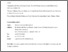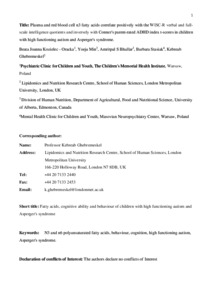# Plasma and red blood cell n3 fatty acids correlate positively with the WISC-R verbal and full-scale intelligence quotients and inversely with Conner's parent-rated ADHD index t-scores in children with high functioning autism and Asperger's syndrome

Kozielec-Oracka, Beata Joanna, Min, Yoeju, Bhullar, Amritpal S., Stasiak, Barbara and Ghebremeskel, Kebreab (2022) Plasma and red blood cell n3 fatty acids correlate positively with the WISC-R verbal and full-scale intelligence quotients and inversely with Conner's parent-rated ADHD index t-scores in children with high functioning autism and Asperger's syndrome. Prostaglandins, leukotrienes, and essential fatty acids, 178 (102414). pp. 1-8. ISSN 1532-2823Preview
Text
FATTY-ACIDS-&-AUTISM_KOZIELEC-ET-AL_6-4-22.pdf - Accepted Version

Official URL: https://doi.org/10.1016/j.plefa.2022.102414

## Abstract / Description

Findings of the fatty acid status of people with autism spectrum disorders have been incongruent perhaps because of the diversity of the condition. A cross-sectional design study was used to  investigated fatty acid levels and relationships between fatty acids, and cognition and behaviour in a homogenous group of children with autism spectrum disorder. Children with Asperger's syndrome (AS) /high functioning autism (n = 44) and healthy siblings (n = 17) were recruited from the Diagnostic and Therapeutic Centre for Children with Autism, Warsaw, Poland. In the AS group, plasma phosphatidylcholine 22:5n3 correlated positively with verbal (r = 0.357, p = 0.019) and full scale (r = 0.402, p = 0.008) IQs, red blood cell phosphatidylcholine 22:5n3 with verbal (r = 0.308, p = 0.044), performance (r = 0.304, p = 0.047) and full scale (r = 0.388, p = 0.011) IQs and red blood cell phosphatidylethanolamine 22:5n3 with verbal (r = 0.390, p = 0.010) and full scale (r = 0.370, p = 0.016) IQs. Whilst, plasma phosphatidycholine 20:5n3 (r = -0.395, p = 0.009), 22:6n3 (r = -0.402, p = 0.007) and total n3 fatty acids (r = -425, p = 0.005), red blood cell phosphatidlycholine 20:5n3 (r = -0.321, p = 0.036) and red blood cell phosphatidylethanolamine 20:5n3 (r = -0.317, p = 0.038), 22:6n3 (r = -0.297, p = 0.05) and total n3 fatty acids (r = -0.306, p = 0.046) correlated inversly with ADHD index. Similarly, inattention was negatively related with plasma phosphatidylcholine 22:6n3 (r = -0.335, p = 0.028), and total n3 fatty acids (r = -0.340, p = 0.026), oppositional with plasma phosphatidylcholine 18:3n3 (r = -0.333, p = 0.029), 20:5n3 (r = -0.365, p = 0.016), total n3 fatty acids (r = -0.293, p < 0.05), red blood cell phosphatidylcholine 18:3n3 (r = -0.337, p = 0.027) and red blood cell ethanolamine 18:3n3 (r = - 0.333, p = 0.029), 20:5n3 (r = -0.328, p = 0.032), 22:6n3 (r = 0.362, p = 0.017) and total n-3 fatty acids (r = -0.298, p < 0.05) and hyperactivity with plasma phosphatidylcholine 22:6n3 (r = -0.320, p = 0.039). In contrast, there were inverse correlations between red blood cell phosphatidylcholine 18:2n6 and performance (r = -0.358, p = 0.019) and full scale (r = -0.320, p = 0.039) IQs, and direct correlations between red blood cell phosphatidylcholine 22:4n6 (r = 0.339, p = 0.026) and 22:5n6 (r = 0.298, p < 0.05) and ADHD index, between red blood cell phosphatidylcholine 22:4n6 (r = 0.308, p = 0.044) and inattention, between plasma phosphatidylcholine 22:4n6 (r = 0.341, p = 0.025), red blood cell phosphatidylcholine 20:4n6 (r = 0.314, p = 0.041) and total n6 fatty acids (r = 0.336, p = 0.028) and oppositional and plasma phosphatidylcholine 20:3n6 (r = 0.362, p = 0.018) and red blood cell phosphatidylcholine 20:3n6 (r = 0.401, p = 0.009) and hyperactivity. The findings of the ethnically homogenous children with Asperger's syndrome/high functioning autism study revealed positive associations between 22:5n3 and cognition, and negative relationships between 20:5n3 and 22:6n3 and behavioural problem. In contrast, cognitive ability and behavioural problems were negatively and positively associated with n6 fatty acids. Further investigation is required to establish whether there a cause and effect relationship. Regardless, it would be prudent to ensure that children with the conditions have optimum n3 PUFA intake.

Item Type: Article N3 and n6 polyunsaturated fatty acids (PUFA); behaviour; cognition; high functioning autism; Asperger’s syndrome 500 Natural Sciences and Mathematics > 570 Life sciences; biology School of Human Sciences Kebreab Ghebremeskel 06 Apr 2022 11:54 06 Apr 2022 11:54 https://repository.londonmet.ac.uk/id/eprint/7385View Item